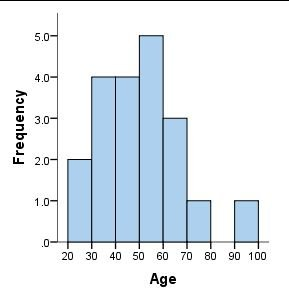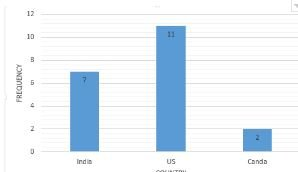# What is the difference between Histogram & Bar Chart?

Standard
 Histogram Bar ChartThe x-axis represents bins. So if you have a continuous variable like age which has values from 0-100 then you can create bins like 0-10, 10-20 and so on (and here bin size = 10). You can change the bin size to analyze the distribution of the data. X-axis has a numerical (quantitative) variable. The x-axis represents distinct categories from your data. The variable on the x-axis is usually qualitative The order of the bins is important since it is used to understand the distribution of the data. The order of the categories in the bar chart doesn’t matter. We can sort it if we want but it’s not needed.